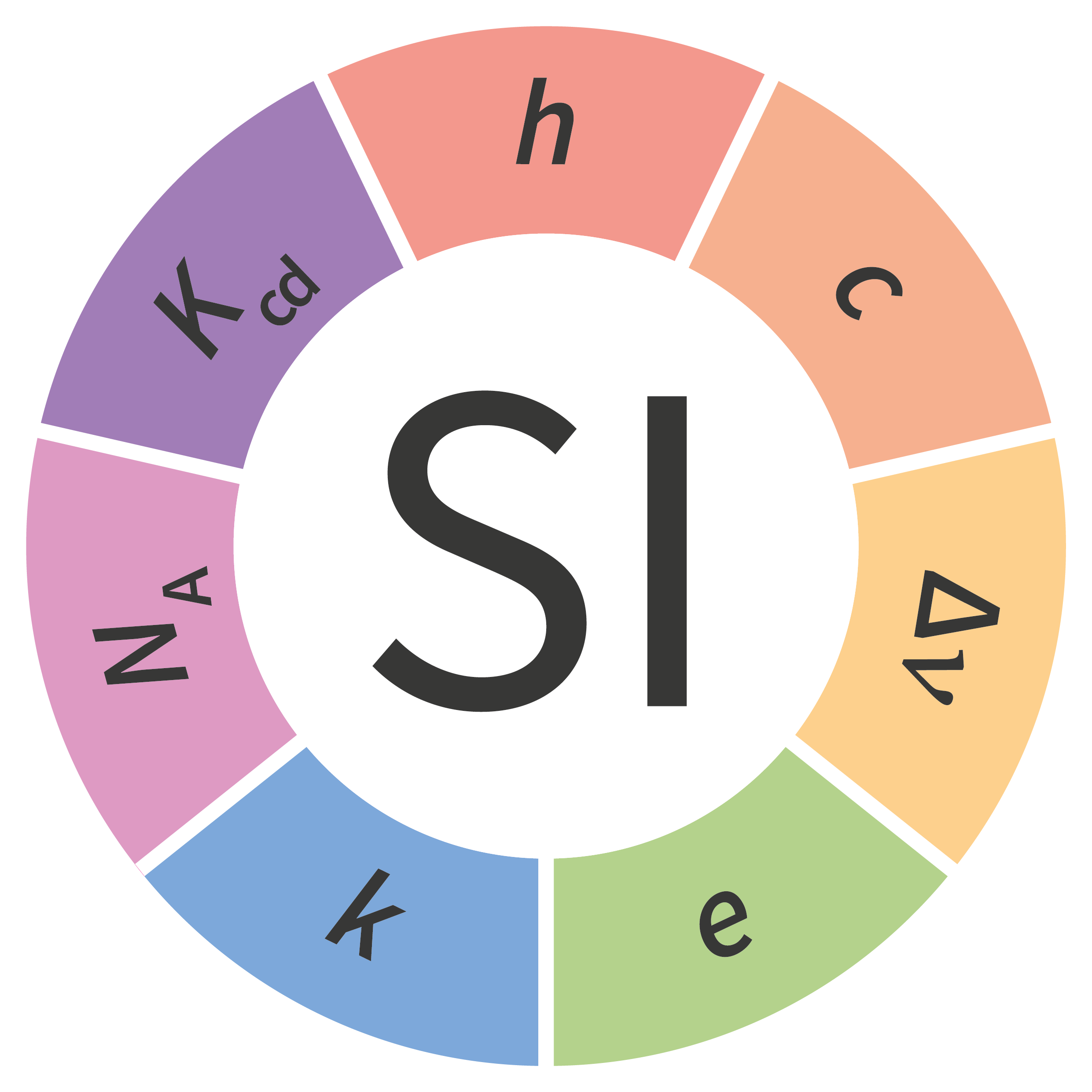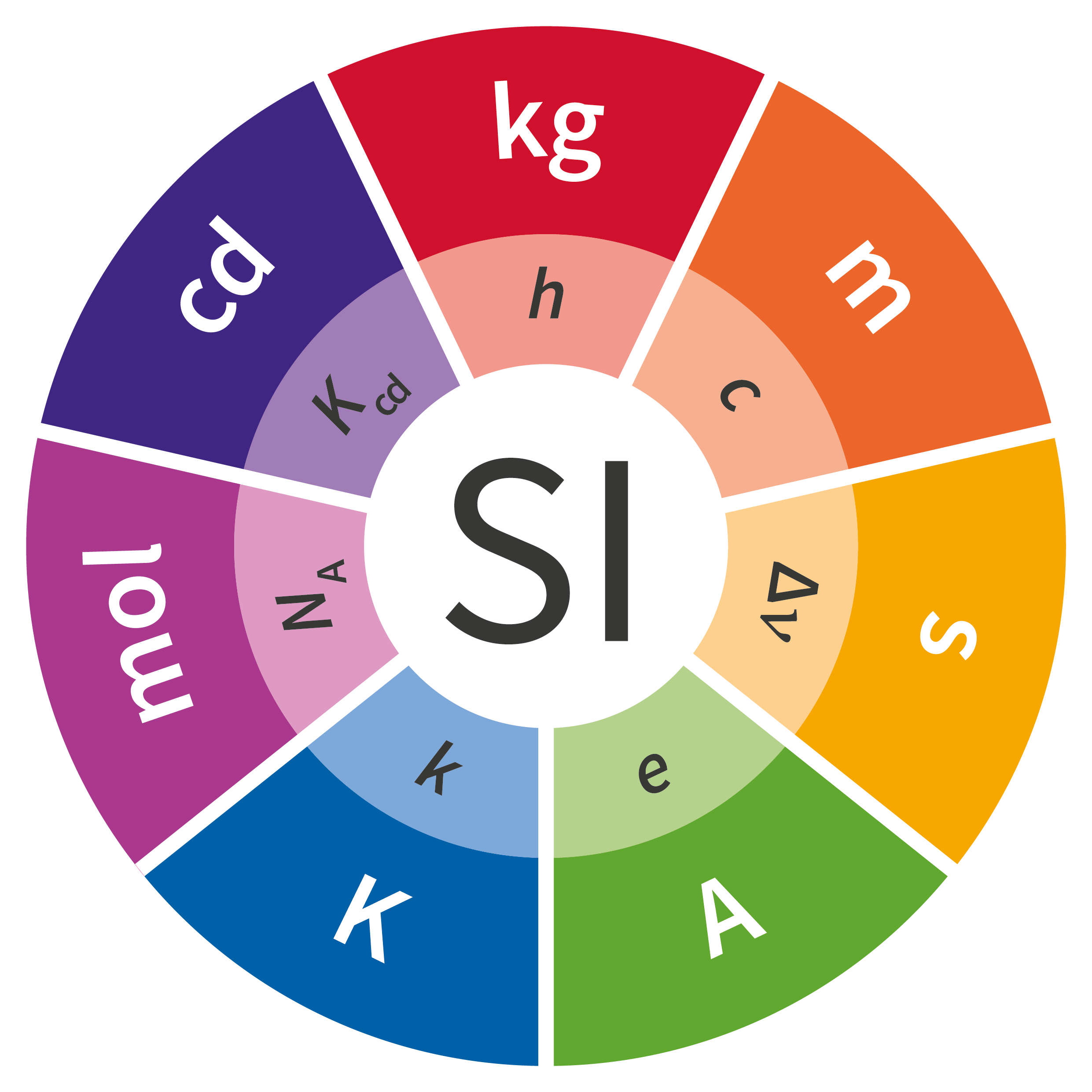# SI units RealisationThe International System of Units, the SI, has been widely accepted around the world as the preferred system of units for science, technology, industry and trade since it was established in 1960 at the 11th meeting of the Conférence Générale des Poids et Mesures, the CGPM (the General Conference on Weights and Measures, in English).

On 20 May 2019, came into force a new approach to articulating the definitions of the SI units by fixing the numerical values of seven “defining” constants.

As a signatory to the Metre Convention, Singapore adopts the redefined SI to ensure that we are consistent with international best practice. The National Metrology Cerntre, A*STAR, is the primary agency responsible for implementing the SI definitions in Singapore.

## Definition of the SI

The International System of Units, the SI, is the system of units in which

• the unperturbed ground state hyperfine transition frequency of the caesium 133 atom ΔνCs is 9 192 631 770 Hz,
• the speed of light in vacuum c is 299 792 458 m/s,
• the Planck constant h is 6.626 070 15 × 1034 J s,
• the elementary charge e is 1.602 176 634 × 1019 C,
• the Boltzmann constant k is 1.380 649 × 1023 J/K,
• the Avogadro constant NA is 6.022 140 76 × 1023 mol1,
• the luminous efficacy of monochromatic radiation of frequency 540 × 1012 Hz, Kcd, is 683 lm/W,

where the units hertz, joule, coulomb, lumen, and watt, with unit symbols Hz, J, C, lm, and W, respectively, are related to the units second, metre, kilogram, ampere, kelvin, mole, and candela, with unit symbols s, m, kg, A, K, mol, and cd, respectively, according to

• Hz = s–1,
• J = kg m2 s–2,
• C = A s,
• lm = cd m2 m–2 = cd sr, and
• W = kg m2 s–3.

The numerical values of the seven defining constants have no uncertainty.

Since the product of the numerical value and the unit has to equal the value of a constant, by fixing the exact numerical value the unit becomes defined. The seven constants are chosen in such a way that any unit of the SI can be written either through a defining constant itself or through products or quotients of defining constants. All SI units are defined in terms of constants that describe the natural world. This will assure the future stability of the SI and open the opportunity for the use of new technologies, including quantum technologies, to implement the definitions.

Prior to the new revision of the SI, SI units have been presented in terms of a set of, most recently seven, base units, namely• the second, s, SI unit of time
• the meter, m, SI unit of length
• the kilogram, kg, SI unit of mass
• the ampere, A, SI unit of electric current
• the kelvin, K, SI unit of thermodynamic temperature
• the mole, mol, SI unit of amount of substance, and
• the candela, cd, SI unit of luminous intensity in a given direction.
• All other units, described as derived units, are constructed as products of powers of the base units. This concept of base and derived units is maintained. With the new revision, the definitions of all the base units can now be deduced by using one or more of these defining constants.

## Definitions of the SI units

1. The second, symbol s, is the SI unit of time. It is defined by taking the fixed numerical value of the caesium frequency ΔνCs, the unperturbed ground-state hyperfine transition frequency of the caesium 133 atom, to be 9 192 631 770 when expressed in the unit Hz, which is equal to s1.2. The metre, symbol m, is the SI unit of length. It is defined by taking the fixed numerical value of the speed of light in vacuum c to be 299 792 458 when expressed in the unit m s1, where the second is defined in terms of the caesium frequency ΔνCs.3. The kilogram, symbol kg, is the SI unit of mass. It is defined by taking the fixed numerical value of the Planck constant h to be 6.626 070 15 × 1034 when expressed in the unit J s, which is equal to kg m2 s1, where the metre and the second are defined in terms of c and ΔνCs.4. The ampere, symbol A, is the SI unit of electric current. It is defined by taking the fixed numerical value of the elementary charge e to be 1.602 176 634 × 10−19 when expressed in the unit C, which is equal to A s, where the second is defined in terms of ΔνCs.5. The kelvin, symbol K, is the SI unit of thermodynamic temperature. It is defined by taking the fixed numerical value of the Boltzmann constant k to be 1.380 649 × 1023 when expressed in the unit J K1, which is equal to kg m2 s2 K1, where the kilogram, metre and second are defined in terms of h, c and ΔνCs.6. The mole, symbol mol, is the SI unit of amount of substance. One mole contains exactly 6.022 140 76 × 1023 elementary entities. This number is the fixed numerical value of the Avogadro constant, NA, when expressed in the unit mol1 and is called the Avogadro number. The amount of substance, symbol n, of a system is a measure of the number of specified elementary entities. An elementary entity may be an atom, a molecule, an ion, an electron, any other particle or specified group of particles.7. The candela, symbol cd, is the SI unit of luminous intensity in a given direction. It is defined by taking the fixed numerical value of the luminous efficacy of monochromatic radiation of frequency 540 × 1012 Hz, Kcd, to be 683 when expressed in the unit lmW−1, which is equal to cd srW−1, or cd sr kg−1 m−2 s3, where the kilogram, metre and second are defined in terms of h, c and ΔνCs.The derived units were constructed as products of powers of the base units. In fact, defining the SI by fixing the numerical values of seven defining constants has the effect that this distinction is, in principle, not needed any more, since all units, base or derived units, can now be written either through a defining constant itself or through products or quotients of defining constants.

The new approach will assure the future stability of the SI and open the opportunity for the use of new technologies, including quantum technologies, to implement the definitions.